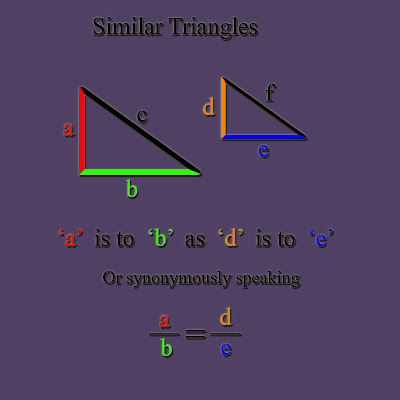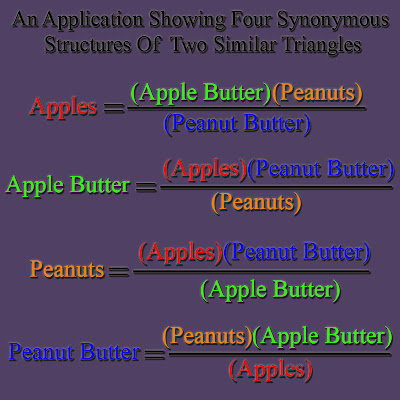## Sunday, April 15, 2007

### Similar Triangles Poems

Similar Triangles Poems / Proportional Poems

I would like to map out the structure and show examples of what I call "Similar Triangles Poems"or "Proportional Poems". Let me use the example of Similar Triangles to help visualize the proportional relationship in this mathematical structure.

Let us first look at two similar triangles with sides labeled ‘a’ ‘b’ ‘c’ and the second with sides labeled ‘d’ ‘e’ and ‘f’ Notice the laws of geometry state that a/b is equal to d/e as shown below. I am going to call this latter equation "The Similar Triangles Relationship".Also let us note that we can solve for any or all of the variables. This will give us four synonymous variations of the similar triangles relationship in terms of one variable – examples shown in the next slide:Now let us look at the logical structure of the following comparison: Apples are to apple butter as peanuts are to peanut butter. Furthermore, let us also look at how we can map the latter statement into the similar triangles relationship.
The following slide shows us a good example of how metaphor can be applied to the relationship of similar triangles.Now let us substitute the terms of our logical comparison into the all of the similar variations to create four similar triangles poems.We now have four poems that are logically equivalent but syntactically different. Each poem says the same thing only with a different flavor much like playing a piece of music in four different keys.

The pedagogical example above uses rather mundane subject matter. To see more poetic examples, please click on the lablel for "Similar Triangles Poems" (below)